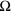# Ohm's Law

Next Page: Power and Energy

Also See: Voltage & Current | Resistance

## Ohm's Law shows the relationship between voltage, current and resistance

To make a current flow through a resistance there must be a voltage across that resistance. Ohm's Law shows the relationship between the three quantities: voltage, current and resistance.

Ohm's Law can be written as a word equation:

 voltage = current × resistance

Or using symbols to represent the quantities of voltage (V), current (I) and resistance (R):

 V = I × R

In fact it can be written three ways and you can pick the version that's best for your purpose:

 V = I × R
 I = V R
 R = V I

## The VIR triangle - a way to remember Ohm's Law

 V I    R

You can use the VIR triangle to help you remember the three versions of Ohm's Law.

• To calculate voltage, V: put your finger over V, this leaves I R, so the equation is V = I × R
• To calculate current, I: put your finger over I, this leaves V over R, so the equation is I = V/R
• To calculate resistance, R: put your finger over R, this leaves V over I, so the equation is R = V/I

## Use the right units

For most electronic circuits the amp is too large and the ohm is too small, so we often measure current in milliamps (mA) and resistance in kilohms (k).

1 mA = 0.001 A
1 k= 1000.

The Ohm's Law equations work if you use V, A and, or if you use V, mA and k.

It is vital to use the right units for the three quantities in Ohm's Law, otherwise calculations will give the wrong values.

You can use either of these two sets of units:

 V = voltage in volts (V)I  = current in amps (A) R = resistance in ohms ()

or

 V = voltage in volts (V)I  = current in milliamps (mA) R = resistance in kilohms (k)

You must not mix these sets of units in the equations so you may need to convert between mA and A or kand.

## Converting units

Convert the units when necessary using these rules:

#### For current:

 1mA = 0.001A
 1A = 1000mA

#### For resistance:

 1k= 10001= 0.001k#### Example unit conversions

35mA = 0.035A

0.2A = 200mA

3mA = 0.003mA

22k= 22000470= 0.47k3.3k= 3300## Ohm's Law Calculations

Use this method to guide you through calculations:

1. Write down the Values, converting units if necessary.
2. Select the Equation you need (use the VIR triangle).
3. Put the Numbers into the equation and calculate the answer.

It should be Very Easy Now! See the examples below:

#### Example 1:

3V is applied across a 6resistor, what is the current?

• Values: V = 3V, I = ?, R = 6• Equation: I = V/R
• Numbers: Current, I = 3/6 = 0.5A

#### Example 2:

A lamp connected to a 6V battery passes a current of 60mA, what is the lamp's resistance?

• Values: V = 6V, I = 60mA, R = ?
• Equation: R = V/I
• Numbers: Resistance, R = 6/60 = 0.1k= 100(using mA for current means the calculation gives resistance in k)

#### Example 3:

A 1.2kresistor passes a current of 0.2A, what is the voltage across it?

• Values: V = ?, I = 0.2A, R = 1.2k= 1200(1.2kis converted to 1200because A and kmust not be used together)
• Equation: V = I × R
• Numbers: V = 0.2 × 1200 = 240V

#### Example 4:

9V is applied across a 15kresistor, what is the current?

• Values: V = 9V, I = ?, R = 15k• Equation: I = V/R
• Numbers: Current, I = 9/15 = 0.6mA
(using kfor resistance means the calculation gives current in mA)

Next Page: Power and Energy | Study